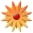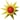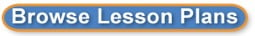# Let's Make Subtraction Movies!

Page Views: 3154

Email This Lesson Plan to Me
Overall Rating:(5.0 stars, 1 ratings)

 Keywords: flip camera, subtraction Subject(s): Math Grade 1 School: Dozier Elementary School, Erath, LA Planned By: Regina Caillouet Original Author: Regina Caillouet, Erath
Subtracting with Flip Cameras!

�Subtraction
�Number sentences (explaining process and modeling it)

Time: One Week

Lesson: Let's Make Subtraction Movies!

1. Review subtraction facts.

2. Introduce subtraction sentences to the students.

3. Model several examples for the students. (Mom had 4 cookies. I ate two. How many are left?

4. Make several classroom number sentences and discuss them as you go.

5. Then create student groups of 3 or 4.

6. Have the students in each group will write subtraction sentences using cereal pieces or colored marshmallows. (Any objects are acceptable.)

7. Once each group has decided on the number sentence the children will write a number sentence and illustrate it.

8. Each group will then go through the process of sharing their number sentences while the teacher (or student in the group) films their explanation.

9. Take digital pictures of each group during the process.

10. The teacher can insert the photos of each group in one document and print as a
Practice activity. The students would write a number sentence near the photo inserted.

11. Finally, the teacher will show the combined problems as one movie after.

Assessment: Teacher observation of the concept taught and look over the completed document with (photos and) number sentences written.
 Cross-Curriculum Ideas Math/Writing Links: Math Fact Page HelpSubtraction Practice Help Materials: Computer Accessories, Flash/USB Drives, Printers, Hard Drives, Point and Shoot, Flip Video, Power, Keyboards, Mice